# BSpline fitting parametrization

Hello guys,

I’m trying to do BSpline fitting to estimate vessel centerlines given some user points.
I use the BSplineScatteredDataPointSetToImageFilter and it gives good results in most cases.
But when the parametric points are almost aligned (~ representing a straight line), the fitting tend to return a very wavy fit that really overestimate the path.
I’ve tried with varying spline order and level parameters but I’ve failed to make it works properly.
For comparison I’ve used the vtk cardinal spline fitting and it seems to give better results, but I don’t understand why.

Here an example of my problem (given points with linear interpolation ; itk fitting ; vtk fitting):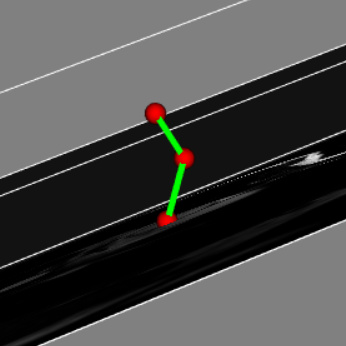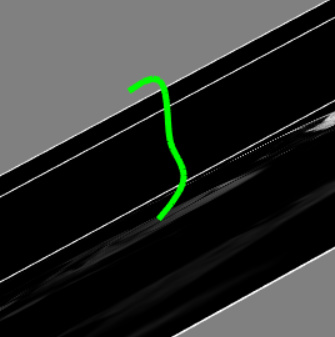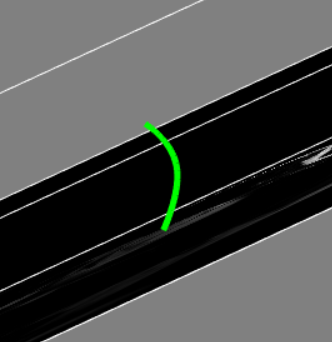Here a working case (given points ; itk fitting):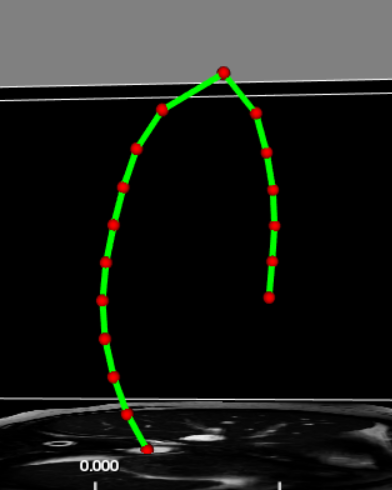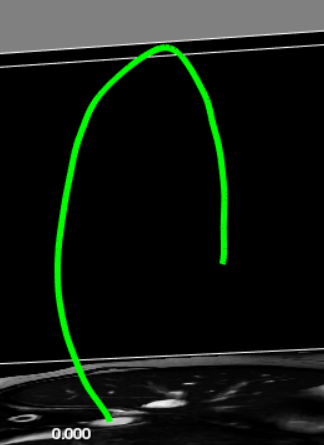Is it an implementation problem or should I be able to get a good fit?

Tim

Hi Tim,

From the screenshots (nice!) it looks like reducing the spline order may help.

The get a curve that is realistic by utiliizing the image content, the ITKMinimalPathExtraction module may be useful.

HTH,
Matt

CC: @ntustison

1 Like

Hi Tim,

How many control points and fitting levels did you specify for the top, middle figure?

Nick

Hello Nick!

This is obtained with 4 controls points and 10 levels.

(@matt.mccormick, I’ve tried reducing the order, but that was unsuccessful)

Tim

For three data points, a cubic spline and 10 fitting levels (starting with four control points) is definitely overkill. Since you’re doubling the spline resolution at each level, you’re ending up with 2^10 = 1024 piecewise splines which is causing the above results.

When I try lowering the level depth and/or the spline order, it worsen the fit (except when going to first order).
This configuration can also give seemingly nice results with few points, as this case, hence my puzzling :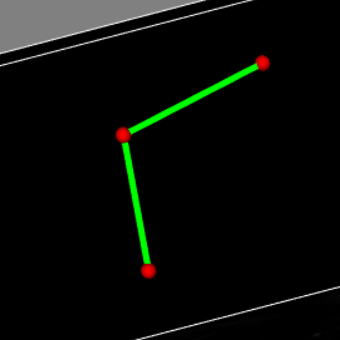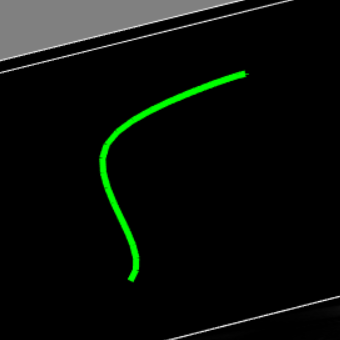I’ll fiddle a bit more with parameters to see if I can achieve a consistent behavior for my needs, before switching to something else.

In any case, many thanks for the hints Nick!

Tim

Another relevant issue of which you need to be aware is something that is particular to this specific fitting approach. The original formulation of Lee et al. (cited in the class) allows one to handle scattered data unlike other approaches (e.g., least squares). The drawback is that the default “fitting value” is zero, or at least that’s the best way to describe it. So you’re going to get a fundamentally different fit if the centroid of the points is zero vs. if the centroid in 3-D space is far from 0. All that being said, when I fit a curve using this class, I subtract the centroid from each data point before fitting and then add that centroid value to all sampled data points after fitting but before using the curve values.

3 Likes

Nick,

This is definitely a good advice: centering the point set has greatly improved my results!Here is the first presented case now.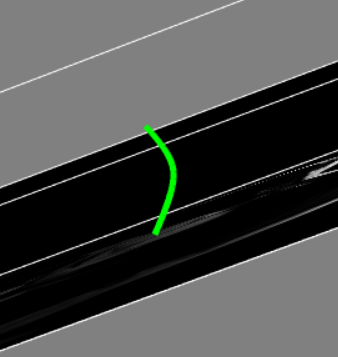Thanks a million for your help.

Tim

2 Likes

Hello folks,

Once again I need your insight on this matter. I’m trying to fit a closed spline based on user input.
From N user input points describing a contour, I want to sample 30 points on a fitted cubic spline.
Thus the spline order is 3 and the number of control points is 4.

When I use the filter in open dimension mode, just adding the first point also as the last one to mimic a closed curve, everything goes fine, but the quality of fitting around starting point is obviously lower due to the absence of continuity constraint.
My problem is two-fold: when I use the filter with closed dimension, the number of control point need to be at least 7, otherwhile an exception is thrown during update due to this part of code.

``````// Generate the control point lattice

typename RealImageType::SizeType size;
for( unsigned int i = 0; i < ImageDimension; i++ )
{
if( this->m_CloseDimension[i] )
{
size[i] = this->m_CurrentNumberOfControlPoints[i] - this->m_SplineOrder[i];
}
else
{
size[i] = this->m_CurrentNumberOfControlPoints[i];
}
}
this->m_PhiLattice = PointDataImageType::New(); // <- with closed dimension size is too low with 4 control points
``````

Is that an expected behavior ? From the guide it was not clear, I understood that it was internally a constraint about first and last control points being equals.

Second part of the problem is the result of fitting with closed dimension is really different, visually much more erroneous, and I don’t understand why. Here is the results of the fitting.
a/ open-mode + 4 control points ;
b/ open-mode + 7 CPs (user points located by yellow circles, and result of linear fitting in green) ;
c/ closed-mode + 7 CPs ;
a/

b/ c/

So my question: does a fitting of closed curve requires specific parametrization that I’m obviously missing ?
I’m adding my fitting code below.

N.B. by the way the interface of the filter for just evaluating curve without image doesn’t seem to be possible anymore ? We can deactivate the image generation but Evaluate method is non existant.

``````      std::vector<itk::Vector<double, 2>> InputPoints(UserSelectedPts1.size());//
for (unsigned int i = 0; i < UserSelectedPts1.size(); i++)
{
itk::Vector<double, 2> tmpVect;
tmpVect = UserSelectedPts1[i];
tmpVect = UserSelectedPts1[i];
InputPoints[i] = tmpVect;
}
// close the contour
{
itk::Vector<double, 2> tmpVect;
tmpVect = UserSelectedPts1;
tmpVect = UserSelectedPts1;
InputPoints.push_back(tmpVect);
}

/* itk fitting take the pointset (proto-mesh) structure as input, where the point id serve the parametric dimension whereas the point data is the data dimension
i.e. to fit a line on 2d point, parametric dimension is 1, data dimension is 2
*/
const unsigned int DataDimension = 2, ParametricDimension = 1;
using ParametricType = itk::Point<float, ParametricDimension>;
using FittingPointSet = itk::PointSet<DataType, ParametricDimension>;

//the bspline filter will output an itk image of ParametricDimension dimension, where pixel are of DataType type
typedef itk::Image<DataType, ParametricDimension> BsplineImageType;
typedef itk::BSplineScatteredDataPointSetToImageFilter<FittingPointSet, BsplineImageType> BSplineFitterType;

//here we define the parametrization of the fitting problem, i.e. the order of points for our line fitting
std::vector<ParametricType> Parametrization(InputPoints.size());
float running_distance = 0;
for (unsigned int i = 0; i < Parametrization.size(); i++)
{
ParametricType pt_p;
if (i!=0)
{
running_distance += sqrt(pow(InputPoints[i] - InputPoints[i - 1], 2) + pow(InputPoints[i] - InputPoints[i - 1], 2));
}
pt_p = running_distance;
Parametrization[i] = pt_p;
}
//running_distance += sqrt(pow(InputPoints - InputPoints[InputPoints.size() - 1], 2) + pow(InputPoints - InputPoints[InputPoints.size() - 1], 2));

/*filling the point set :
we center the points set as the fitting method is sensitive to absolute coordinates
i.e. gives differents results depending on how far from origin (0,0,0) are the parametric points
A better fitting is usually obtained near the origin
*/
FittingPointSet::Pointer PointSet = FittingPointSet::New();
itk::Point<double, 2> Centroid;
Centroid = Centroid = 0;
for (unsigned int i = 0; i < InputPoints.size()-1; i++)
{
Centroid += InputPoints[i];
Centroid += InputPoints[i];
}
Centroid /= InputPoints.size()-1;
Centroid /= InputPoints.size()-1;

for (unsigned int i = 0; i < InputPoints.size(); i++)
{
PointSet->SetPoint(i, Parametrization[i]);
itk::Vector<double, 2> CenteredPoint;
CenteredPoint = InputPoints[i] - Centroid;
CenteredPoint = InputPoints[i] - Centroid;
PointSet->SetPointData(i, CenteredPoint);
}

/* Configuration of the desired spline : use an usual cubic spline (as this minimize the curvature of the fitting)
*/
unsigned int SplineOrder = 3, NbOfLevels = 10;
itk::FixedArray<unsigned int, ParametricDimension> NbControlPoints, ClosedDimension;
NbControlPoints = SplineOrder*2 + 1;
ClosedDimension = 0; //this array is to indicate whether the associated dimension is closed/periodic (1) or not (0)

itk::Size<1> BsplineImageSize;
BsplineImageSize = 31;// 30 points give a out-of-domain exception error -> due to arc length approx by euclidean distance ?
itk::Vector<double, 1> BsplineImageSpacing; //the spacing of output image control in fact the sampling of the continuous spline
BsplineImageSpacing = running_distance/30; //i.e. the arc length needed for regularly spaced 30 points
itk::Point<double, 1> BsplineImageOrigin;
BsplineImageOrigin = 0; //start directly at first input point;

/* Setup and run filter */
BSplineFitterType::Pointer SplineFitter = BSplineFitterType::New();
SplineFitter->SetInput(PointSet);
SplineFitter->SetBSplineEpsilon(1e-3);
SplineFitter->SetSplineOrder(SplineOrder);
SplineFitter->SetNumberOfControlPoints(NbControlPoints);
SplineFitter->SetCloseDimension(ClosedDimension);
SplineFitter->SetNumberOfLevels(NbOfLevels);
SplineFitter->SetOrigin(BsplineImageOrigin);
SplineFitter->SetSpacing(BsplineImageSpacing);
SplineFitter->SetSize(BsplineImageSize);
try
{
SplineFitter->Update();
}
catch (itk::ExceptionObject & err)
{
std::cerr << err;
throw;
}
catch (...)
{
throw Exception("ERROR: Unknown error when trying to run the fitting filter\n");
}

std::vector<itk::ContinuousIndex<double, 2>> ReturnedCI;
itk::ImageRegionConstIterator<BsplineImageType> Parser(SplineFitter->GetOutput(), SplineFitter->GetOutput()->GetLargestPossibleRegion());
while (!Parser.IsAtEnd())
{
itk::Vector<double, 2> CenteredPt = Parser.Get();
itk::ContinuousIndex<double, 2> RetPt;
RetPt = CenteredPt + Centroid;
RetPt = CenteredPt + Centroid;
ReturnedCI.push_back(RetPt);
++Parser;
}
ReturnedCI.pop_back();
``````
1 Like